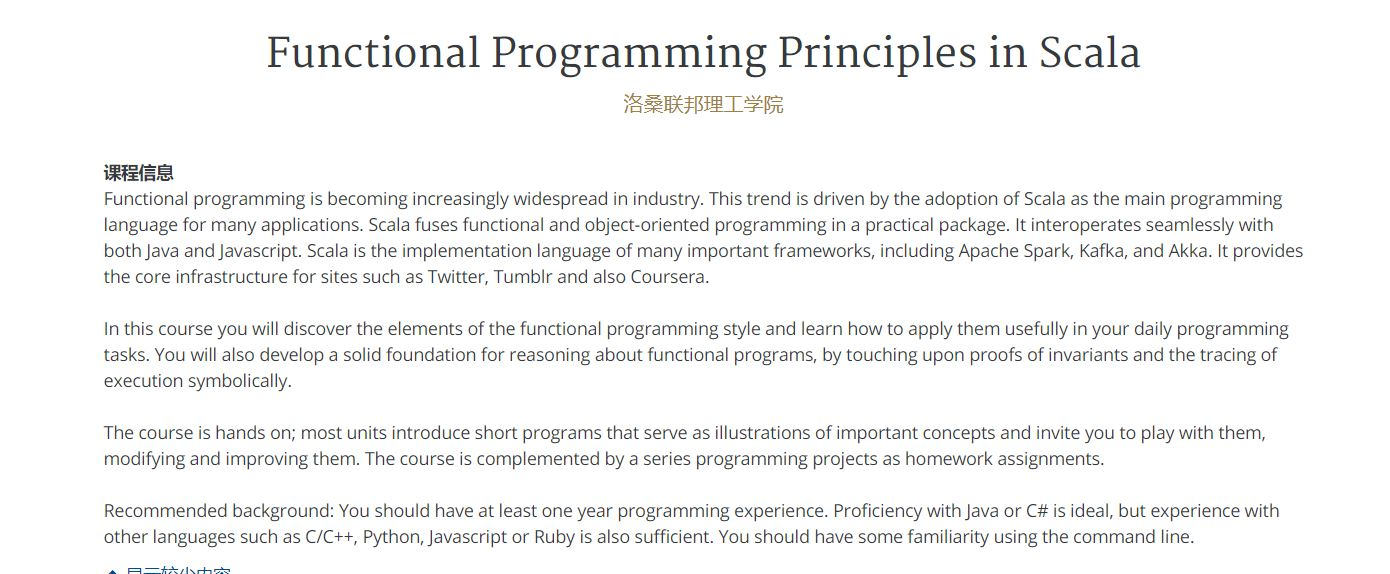## 前言

Scala Puzzle系列要暂时停更一段时间了，之前我以为Scala Puzzle是一本趣味读物，结果发现是进阶读物，做到一半发现做不下去了（摔

## Week 1: Getting Start , Function & Evaluation

### 1.2 Element of Programming

• call by value
• call by name

call by value的好处是对于每一个函数的参数，只用进行一次计算

call by name的好处是对于函数体内没有使用到的参数不用进行计算

• test(2,3)
• test(3+4,8)
• test(7,2*4)
• test(3+4,2*4)

call by value :

• test(2,3) = 2 * 2 = 4

call by name:

• test(2,3) = 2 * 2 = 4

call by value:

• test(3+4,8) = test(7, 8) = 7 * 7 = 49

call by name:

• test(3+4,8) = (3+4) (3+4) = 7 (3+4) = 7 * 7 = 49

call by value:

• test(7,2 4) = test(7,8) = 7 7 =49

call by name:

• test(7,2 4) = 7 7 = 49

call by value:

• test(3+4, 2 4) = test(7, 2 4) = test(7, 8) = 7 * 7 = 49

call by name:

• test(3+4, 2 4) = (3+4) (3+4) = 7 (3+4) = 7 7 = 49

### 1.3 Evaluation Strategies and Termination

y: => Int这样的写法，是为了指明对于参数y用call by name的方式来使用

• constOne(1+2, loop)
• constOne(loop, 1+2)

constOne(1+2, loop) :

• constOne(1+2, loop) = constOne(3, loop) = 1

constOne(loop, 1+2):

• constOne(loop, 1+2) = constOne(loop, 1+2) = ....

### 1.5 Example: square roots with Newton's method

1. isGoodEnough函数对于非常小的数字不太准确，对于很大的数字看起来又无法终止，能解释原因吗
2. 重新实现isGoodEnough函数以规避上述问题
3. 测试以下样例
• 0.0001
• 0.1e-20
• 1.0e20
• 1.0e50
1. 对比较小的数据不准确是因为isGoodEnough用的是绝对误差,可对该函数做简单修改，以输出中间值

运行结果为

这里 0.03 * 0.03 - 0.0001 < 0.001但是显然最后的结果不好

我们当然可以直接把0.001改成一个更小的值，但更好的一个做法是用相对误差，修改后的函数如下

修改后的结果看起来更正常了

对应地，对于较大的输入的数，它根本不会收敛到终止条件。

这是因为double 类型浮点数，在IEEE 754标准中，用64位来存储，其中分配了52位来存储浮点数的有效数字，11位存储指数，1位存储正负号

在二进制表示中，有效数字大于52位的部分会舍去

这里可以看到1e60转换成二进制有近166位，而浮点数的精度只有52位，也就是说，最小误差也是2^114远大于0.001,所以自然不会到达终止条件

2. 基于以上的分析，我们重新实现的函数如下

3. 运行测试

### Example Assignment

Notes: 注意不要修改已经实现的方法或名称

submit [email protected] HEmio6RWZ7ribfh1

### 作业： Recursion

1. 帕斯卡三角（杨辉三角）

pascal(0,2) = 1, pascal(1,2) = 2, pascal(1,3) = 3

求paccal(col, row)的值，col和row都是从0开始计算

如果你还记得二次项系数（二项式定理），那么就好办了
$$若 C^m_n = \frac{n!m!}{(n-m)!} ,n >= m 且 m,n∈N^+$$
那么有
$$C_n^m = C_{n-1}^{m-1} + C_n^{m-1}$$

$$C_n^0 = C_n^n = 1$$

1. 判断括号是否平衡

判断一串字符串文本中包含的括号是否“平衡”

例如，以下序列为平衡

• (if (zero? x) max (/ 1 x))
• I told him (that it’s not (yet) done). (But he wasn’t listening)

以下序列为不平衡

• :-)
• ())(

简单来说 可以总结为如下规则

• 空串或者不含括号的字符串为平衡的
• 如果R为平衡的，那么(R)也是平衡的
• 如果R为平衡的，S为平衡的，那么RS也是平衡的

代码实现如下

思路是，一个平衡的序列，()数量必须相同且合法。

• 空串是合法的序列
• 如果字符串的第一个字符为(， 当前计数+1，递归执行剩下的字符串
• 如果字符串的第一个字符为)， 且当前计数大于零，那么递归执行剩下的字符串，当前计数-1
• 如果不符合以上条件，递归执行剩下的字符串
2. 计算找零

给你一定数目的钱money，问用coins（一个整型数组）中的零钱，有多少种方法能凑够

例如，对于money = 4 , coins = List(1, 2)

有3种方法（交换顺序算一种方法）

4 = 1 + 1 + 1 + 1

= 1 + 1 + 2

= 2 + 2

• 显然，当money = 0 时，结果为0

• 当 money != 0时，设当前的答案为f(money, coins)

那么 f(money, coins) = f(money - coins.head, conins) + f(money, coins.tail)

即当前状态的结果，为用零钱里第一种面额的找零方案，加上不用零钱里第一种面额的找零方案，这两个结果的数目之和

易知这个划分是互不相交且并集为完整结果集

• 再考虑到边界条件

代码实现如下